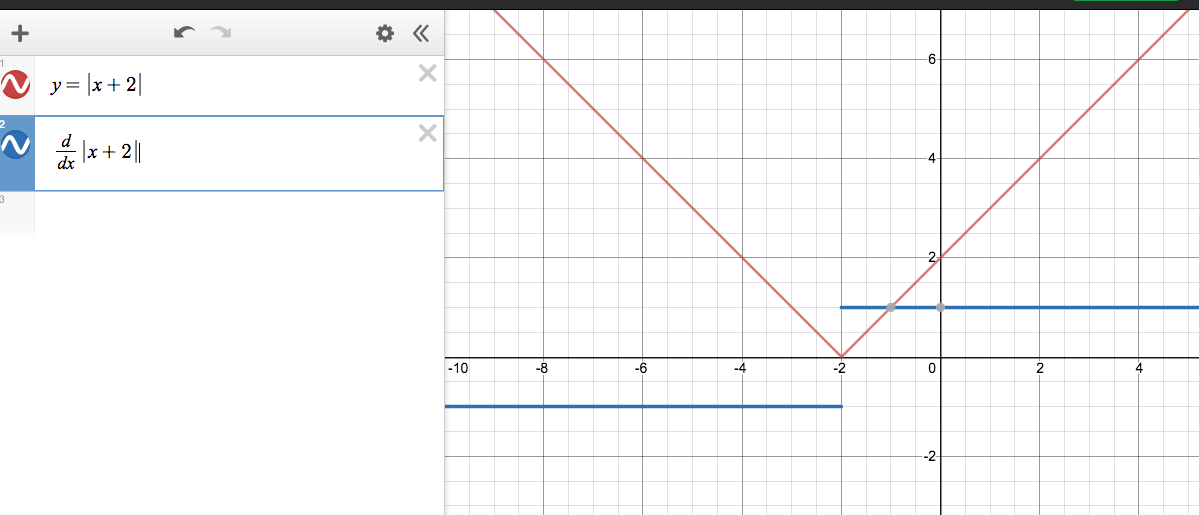Posted on

# Derivatives of Absolute Values

Remember absolute values are really two functions. For example, if |x + 2| = 3, then we find both solutions by solving x + 2 = 3 and x + 2 = -3 (put x = 1 and x = -5 to the original equation to check yourself).

This is complicated, so it’s worthwhile to specifically consider linear functions. The derivative is the rate of change. For linear equation, the rate of change is the slope. So, if we need to take the derivative of y =|x + 2|, then we can see the slope is 1 when x greater than -2. The slope is -1 when x is less than -2.The graph of y = |x+2| has two slopes. The blue line shows the graph of the derivative for each x-value (note that the function is not differentiable at x=-2).

## Video Overview

This video shows how to find derivatives of linear and quadratic absolute functions.